## Basic Math Operations

For more advanced to even the simplest of programs, all your computer is doing at its simplest form, is adding, subtracting, dividing, and multiplying. All things you’ve already learned in primary school, all you need to do is adapt that logic to programming and you're set. We’ll be using a fresh console program for this, so go create a new file. You can name it “mathOpEx” or something along those lines. Math operations can only be run on two variables, Int and Doubles. Since for each of the operations it’s the same exact thing, I will go through the wireframe of it with examples for adding and explain what to do if you’re using other operations.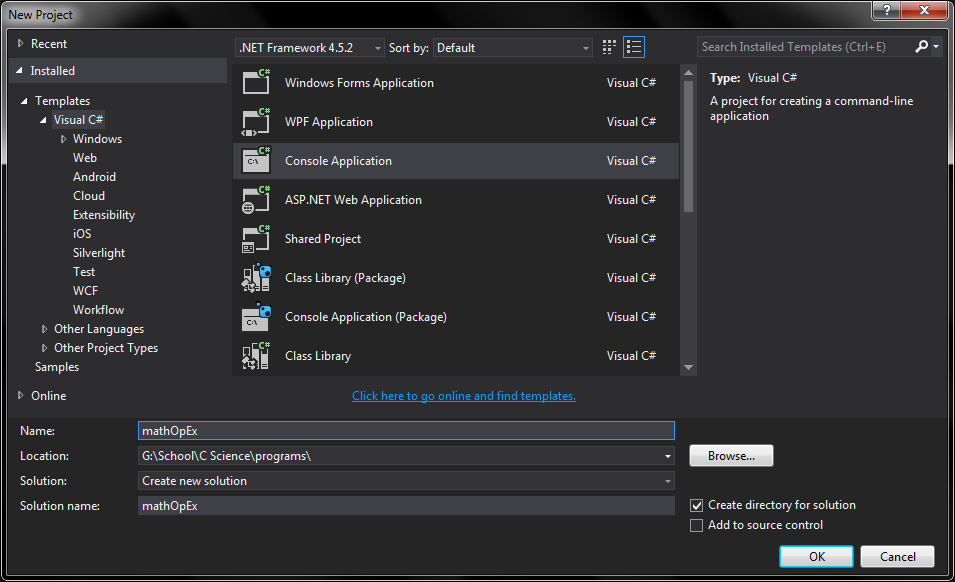First off, we need to declare and initialize our two values that we will be adding, subtracting, multiplying, or dividing depending on what operation you’re using. To do this, declare two Int variables with the labels “I” and “x”. Then, give your variables value. Give “i” a value of 40 and “x” a value of 20. It should look a little something like this, 'Int32 i = 40; / Int32 x = 20;'. Note – the slash represents a line break.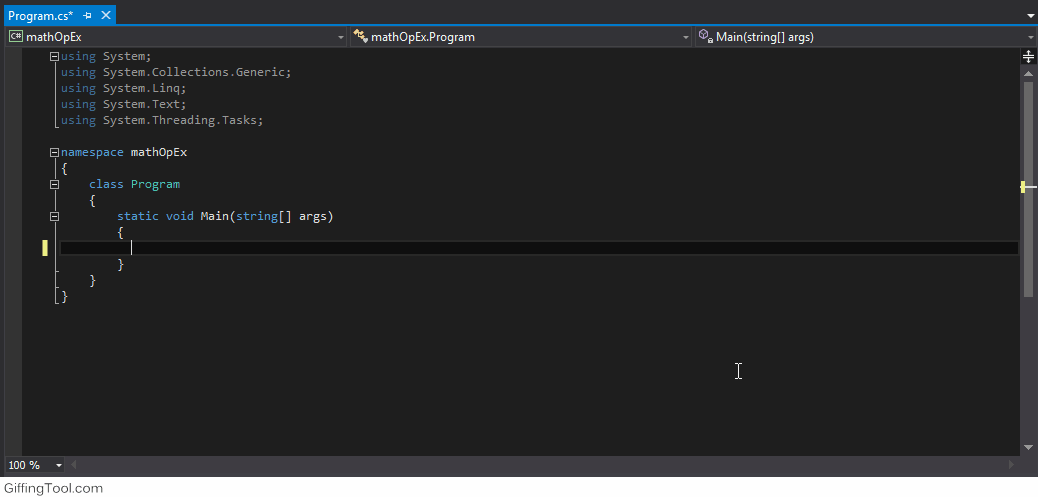Now, we need to create a variable for the operation you are using. For an adding equation, type 'Int32 adding; / adding = i + x;' This will create an integer variable with the name of the operation, and then it will state the variable and give it a parameter. In this case, it’s adding the value of i to x. If you are using another operation, replace the variable name with the name of that operation and then replace the operation symbol with the corresponding one you’re trying to use.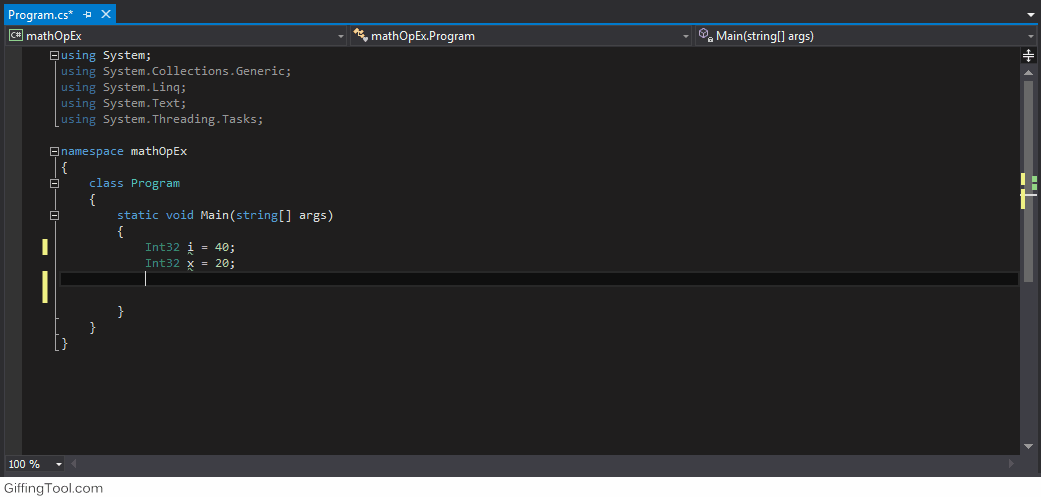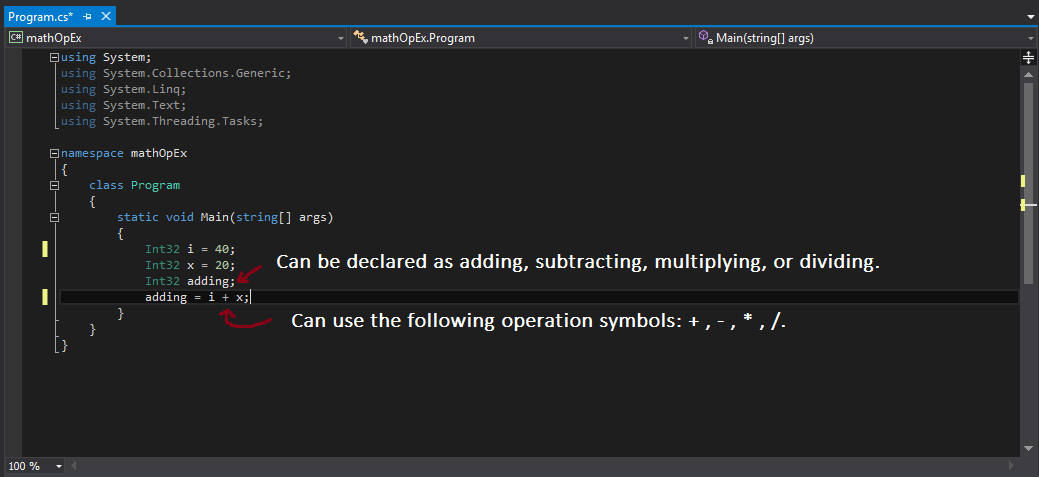This particular line of code is the most important, it takes the operation variable and makes it calculate the values using that formula. Then it takes the x and i values and the total, converts those to strings, and displays them on the screen as an equation. It is written like this ‘Console.WriteLine(String.Concat(i.ToString(), "+", x.ToString(), "=", adding.ToString()));’. To change this code to make it so that it works for your operation, is, you take out the “+” and replace it with whatever operation symbol you need for the command. Then, you change “adding” to whatever name your formula variable is named. Whether it be adding, subtracting, multiplying, or dividing. To finish the program off, create a new line under that and type ‘Console.ReadKey();’. This keeps the console open when you start it.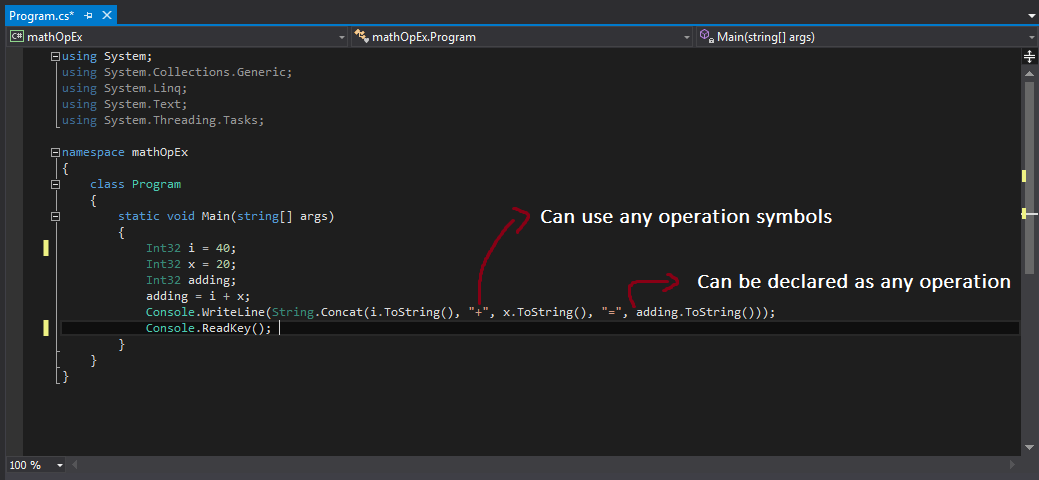You've just learned how to use basic math and math operations in C#. The last section of this guide will be going over how to add strings together in sequence.